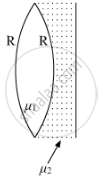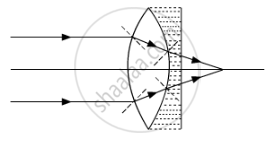# Find the Equivalent Focal Length of the Combination - Physics

A biconvex lens with its two faces of equal radius of curvature R is made of a transparent medium of refractive index μ1. It is kept in contact with a medium of refractive index μ2 as shown in the figure.(a) Find the equivalent focal length of the combination.
(b) Obtain the condition when this combination acts as a diverging lens.
(c) Draw the ray diagram for the case μ1 > (μ2 + 1) / 2, when the object is kept far away from the lens. Point out the nature of the image formed by the system.

#### Solution

From the lens maker formula, we have

1/f=(μ−1)(1/R_1−1/R_2)

where  f=Focal length the lens

μ=Refractive index of material

R1=Radius of curvature of first face

R2=Radius of curvature of second face

Let f1 anf f2 be the focal lengths of the two mediums. Then,

1/f_1=(μ_1−1)[1/R−(−1/R)]

⇒1/f=(μ_1−1)(2/R)1/f_2

=(μ_2−1)[(−1/R)−1/∞]

⇒1/f_2=(μ_2−1)(−1/R)

(a) If feq is the equivalent focal length of the combination, then

1/f_eq=1/f_1+1/f_2

⇒1/f_eq=(2(μ_1−1))/R−(μ_2−1)/R

⇒1/f_eq=(2μ_1−μ_2−1)/R

⇒f_eq=R/(2μ_1−μ_2−1)

(b) For the combination to behave as a diverging lens, feq < 0

⇒R/(2μ_1−μ_2−1)<0

⇒2μ_1−μ_2−1<0

⇒μ_1<(μ_2+1)/2

which is the required condition

(c) For μ1>(μ2+1)/2, the combination will behave as the converging lens. So, an object placed far away from the lens will form image at the focus of the lens.The image so formed will be real and diminished in nature.

Concept: Magnification
Is there an error in this question or solution?
2014-2015 (March) Patna Set 2

Share